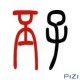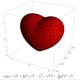# 谬证大全：1+1≠2的n种可能

最近看到几个有趣的数学谬证，想写下来与大家分享；结果写到这个又想到那个，一写就写个没完，于是想到干脆做一篇谬证大全，收集各种荒谬的证明。
如果你有什么更棒的“证明”，欢迎来信与我分享，我会更新到这篇日志中。我的邮箱是 matrix67 at tom.com ，或者 gs.matrix67 at gmail.com 。

1=2？史上最经典的“证明”

设 a = b ，则 a·b = a^2 ，等号两边同时减去 b^2 就有 a·b – b^2 = a^2 – b^2 。注意，这个等式的左边可以提出一个 b ，右边是一个平方差，于是有 b·(a – b) = (a + b)(a – b) 。约掉 (a – b) 有 b = a + b 。然而 a = b ，因此 b = b + b ，也即 b = 2b 。约掉 b ，得 1 = 2 。

这可能是有史以来最经典的谬证了。 Ted Chiang 在他的短篇科幻小说 Division by Zero 中写到：

There is a well-known “proof” that demonstrates that one equals two. It begins with some definitions: “Let a = 1; let b = 1.” It ends with the conclusion “a = 2a,” that is, one equals two. Hidden inconspicuously in the middle is a division by zero, and at that point the proof has stepped off the brink, making all rules null and void. Permitting division by zero allows one to prove not only that one and two are equal, but that any two numbers at all—real or imaginary, rational or irrational—are equal.

这个证明的问题所在想必大家都已经很清楚了：等号两边是不能同时除以 a – b 的，因为我们假设了 a = b ，也就是说 a – b 是等于 0 的。

小学时，这个问题困扰了我很久：下面这个式子等于多少？

1 + (-1) + 1 + (-1) + 1 + (-1) + …

一方面，

1 + (-1) + 1 + (-1) + 1 + (-1) + …
= [1 + (-1)] + [1 + (-1)] + [1 + (-1)] + …
= 0 + 0 + 0 + …
= 0

另一方面，

1 + (-1) + 1 + (-1) + 1 + (-1) + …
= 1 + [(-1) + 1] + [(-1) + 1] + [(-1) + …
= 1 + 0 + 0 + 0 + …
= 1

这岂不是说明 0 = 1 吗？

后来我又知道了，这个式子还可以等于 1/2 。不妨设 S = 1 + (-1) + 1 + (-1) + … ， 于是有 S = 1 – S ，解得 S = 1/2 。

学习了微积分之后，我终于明白了，这个无穷级数是发散的，它没有一个所谓的“和”。无穷个数相加的结果是多少，这个是需要定义的。

同样的戏法可以变出更多不可思议的东西。例如，令

x = 1 + 2 + 4 + 8 + 16 + …

则有

2x = 2 + 4 + 8 + 16 + …

于是

2x – x = x = (2 + 4 + 8 + 16 + …) – (1 + 2 + 4 + 8 + 16 + …) = -1

也就是说

1 + 2 + 4 + 8 + 16 + … = -1

定理：所有数都相等。
证明：取任意两个数 a 和 b ，令 t = a + b 。于是，

a + b = t
(a + b)(a – b) = t(a – b)
a^2 – b^2 = t·a – t·b
a^2 – t·a = b^2 – t·b
a^2 – t·a + (t^2)/4 = b^2 – t·b + (t^2)/4
(a – t/2)^2 = (b – t/2)^2
a – t/2 = b – t/2
a = b

怎么回事儿？

问题出在倒数第二行。
永远记住， x^2 = y^2 并不能推出 x = y ，只能推出 x = ±y 。

1 = √1 = √(-1)(-1) = √-1·√-1 = -1

嗯？

只有 x 、 y 都是正数时， √x·y = √x·√y 才是成立的。
-1 的平方根有两个， i 和 -i 。 √(-1)(-1) 展开后应该写作 i·(-i) ，它正好等于 1 。

考虑方程

x^2 + x + 1 = 0

移项有

x^2 = – x – 1

等式两边同时除以 x ，有

x = – 1 – 1/x

把上式代入原式中，有

x^2 + (-1 – 1/x) + 1 = 0

即

x^2 – 1/x = 0

即

x^3 = 1

也就是说 x = 1。

把 x = 1 代回原式，得到 1^2 + 1 + 1 = 0 。也就是说， 3 = 0 ，嘿嘿！

其实， x = 1 并不是方程 x^2 + x + 1 = 0 的解。在实数范围内，方程 x^2 + x + 1 = 0 是没有解的，但在复数范围内有两个解。
另一方面， x = 1 只是 x^3 = 1 的其中一个解。 x^3 = 1 其实一共有三个解，只不过另外两个解是复数范围内的。考虑方程 x^3 – 1 = (x – 1)(x^2 + x + 1) = 0 ，容易看出 x^3 = 1 的两个复数解正好就是 x^2 + x + 1 的两个解。因此， x^2 + x + 1 = 0 与 x^3 = 1 同时成立并无矛盾。
注意，一旦引入复数后，这个谬论才有了一个完整而漂亮的解释。或许这也说明了引入复数概念的必要性吧。

众所周知，

1 + 2 + 3 + … + n = n(n+1) / 2

让我们用 n – 1 去替换 n ，可得

1 + 2 + 3 + … + (n-1) = (n-1)n / 2

等式两边同时加 1 ，得：

1 + 2 + 3 + … + n = (n-1)n / 2 + 1

也就是

n(n+1) / 2 = (n-1)n / 2 + 1

展开后有

n^2 / 2 + n / 2 = n^2 / 2 – n / 2 + 1

可以看到 n = 1 是这个方程的唯一解。
也就是说⋯⋯ 1 + 2 + 3 + … + n = n(n+1) / 2 仅在 n = 1 时才成立！

这个推理过程中出现了一个非常隐蔽而搞笑的错误。等式两边同时加 1 后，等式左边得到的应该是

1 + 2 + 3 + … + (n-2) + (n-1) + 1

1 块钱等于 1 分钱？

我要用数学的力量掏空你的钱包！请看：

1 元 = 100 分 = (10 分)^2 = (0.1 元)^2 = 0.01 元 = 1 分

用这个来骗小孩子们简直是屡试不爽，因为小学（甚至中学）教育忽视了一个很重要的思想：单位也是要参与运算的。事实上， “100 分 = (10 分)^2” 是不成立的， “10 分” 的平方应该是 “100 平方分” ，正如 “10 米” 的平方是 “100 平方米” 一样。

下面这个“证明”是由数学家 George Pólya 给出的：任意给定 n 匹马，可以证明这 n 匹马的颜色都相同。
对 n 施归纳：首先，当 n = 1 时命题显然成立。若命题对 n = k 成立，则考虑 n = k + 1 的情形：由于 {#1, #2, …, #k} 这 k 匹马的颜色相同， {#2, #3, …, #k+1 } 这 k 匹马也相同，而这两组马是有重叠的，可知这 k+1 匹马的颜色也都相同了。

这个证明错在，从 n = 1 推不出 n = 2 ，虽然当 n 更大的时候，这个归纳是正确的。这是数学归纳法出错的一个比较奇特的例子：基础情形和归纳推理都没啥问题，偏偏卡在归纳过程中的某一步上。

下面，我来给大家证明，所有正整数都相等。
为了证明这一点，只需要说明对于任意两个正整数 a 、 b ，都有 a = b 。
为了证明这一点，只需要说明对于所有正整数 n ，如果 max(a, b) = n ，那么 a = b 。
我们对 n 施归纳。当 n = 1 时，由于 a 、 b 都是正整数，因此 a 、 b 必须都等于 1 ，所以说 a = b 。若当 n = k 时命题也成立，现在假设 max(a, b) = k + 1 。则 max(a – 1, b – 1) = k ，由归纳假设知 a – 1 = b – 1 ，即 a = b 。

这个问题出在， a – 1 或者 b – 1 有可能不是正整数了，因此不能套用归纳假设。

1 是最大的正整数？

来自网友 boring David 发来的邮件：
证明： 1 是最大的正整数。假设最大的正整数不是 1 ，是 a ，则必有 a > 1 。于是有 a^2 > a ，即 a^2 是一个比 a 更大的正整数，与 a 的最大性矛盾。因此 1 是最大的正整数。

这个证明是错误的。在假设最大正整数是 a 之前，你得先说明它的存在性，排除最大的正整数根本不存在的可能性（而事实情况正是后者）。

别以为谬证都是隐藏在数字和字母之中的。下面就是一个经典的几何谬论。
画一个任意三角形 ABC 。下面我将证明， AB = AC ，从而说明所有三角形都是等腰三角形。令 BC 的中垂线与 ∠A 的角平分线交于点 P 。过 P 作 AB 、 AC 的垂线，垂足分别是 E 、 F 。由于 AP 是角平分线，因此 P 到两边的距离相等，即 PE = PF 。于是，由 AAS 可知 △APE ≌ △APF 。由于 DP 是中垂线，因此 P 到 B 、 C 的距离相等，由 SSS 可知 △BPD ≌ △CPD 。另外，由于 PE = PF ， PB = PC ，且 ∠BEP = ∠CFP = 90° ，由 HL 可知 △BEP ≌ △CFP 。现在，由第一对全等三角形知 AE = AF ，由最后一对全等三角形知 BE = CF ，因此 AE + BE = AF + CF ，即 AB = AC 。这个证明过程其实字字据理，并无破绽。证明的问题出在一个你完全没有意识到的地方——这个图形就是错的！事实上， BC 的中垂线与 ∠A 的角平分线不可能交于三角形的内部。我们可以证明， P 点总是落在 △ABC 的外接圆上。如图， P 是 BC 的中垂线与外接圆的交点，显然 P 就是弧 BC 的中点，即弧 BP = 弧 PC 。因此， ∠BAP = ∠CAP ，换句话说 P 恰好就在 ∠A 的角平分线上。P 在 △ABC 外的话，会对我们的证明产生什么影响呢？你会发现，垂足的位置发生了本质上的变化—— F 跑到 AC 外面去了！也就是说，结论 AE + BE = AF + CF 并不错，只是 AF + CF 并不等于 AC 罢了。

下面这个勾股定理的“证明”曾经发表在 1896 年的 The American Mathematical Monthly 杂志上：假设勾股定理是正确的，于是我们可以得到

AB^2 = AC^2 + BC^2
BC^2 = CD^2 + BD^2
AC^2 = AD^2 + CD^2

把后两式代入第一个式子，有

AB^2 = AD^2 + 2·CD^2 + BD^2

但 CD^2 = AD·BD ，因此

AB^2 = AD^2 + 2·AD·BD + BD^2

即

AB^2 = (AD + BD)^2

即

AB = AD + BD

而这显然成立。因此，我们的假设也是成立的。

这个证明是错误的。假设结论正确，推出一个矛盾，确实能说明这个假设是错误的（这就是反证法）；但假设结论正确，推出它与条件吻合，这却并不能说明假设真的就是正确的。错误的假设也有可能推出正确的结果来。最经典的例子就是，不妨假设 1 = 2 ，由等式的对称性可知 2 = 1 ，等量加等量有 1+2 = 2+1 ，即 3 = 3 。但 3 = 3 是对的并不能表明 1 = 2 是对的。

下面这个有趣的故事来源于 Lewis Carroll 的一篇题为 A Logical Paradox 的小论文。
Joe 去理发店理发。理发店有 A 、 B 、 C 三位师傅，但他们并不总是待在理发店里。 Joe 最喜欢 C 的手艺，他希望此时 C 在理发店里。他远远地看见理发店还开着，说明里面至少有一位师傅。另外， A 是一个胆小鬼，没有 B 陪着的话 A 从不离开理发店。
Joe 推出了这么一个结论： C 必然在理发店内。让我们来看看他的推理过程。
反证，假设 C 不在理发店。这样的话，如果 A 也不在理发店，那么 B 就必须在店里了，因为店里至少有一个人；然而，如果 A 不在理发店， B 也理应不在理发店，因为没有 B 陪着的话 A 是不会离开理发店的。因此，由 “C 不在理发店” 同时推出了 “若 A 不在则 B 一定在” 和 “若 A 不在则 B 也一定不在” 两个矛盾的结论。这说明， “C 不在理发店” 的假设是错误的。

从已有的条件看， C 当然有可能不在理发店。但是，为什么 Joe 竟然证出了 C 一定在理发店呢？因为他的证明是错的。其实， “若 A 不在则 B 一定在” 和 “若 A 不在则 B 也一定不在” 并不矛盾——如果事实上 A 在理发店，那么这两个条件判断句都是真的。 “若 A 不在则 B 一定在” 真正的否定形式应该是 “A 不在并且 B 也不在” 。

我曾在 另类搞笑：自我指涉例句不完全收集 一文中写过：

当然，这个定理明显是错的，因为 20 个汉字的组合是有限的，而数是无限多的。这个证明错在哪儿了呢？我也没办法一针见血地道出个所以然来，大家一起来讨论吧。

有趣的是，我们有一个与之相关的（正确的）定理：存在一个实数，它不能用有限个汉字来表达。这是因为，有限长的汉字字符串是可数的，而实数是不可数的。更有趣的是，这个定理的证明必然是非构造性的。

取一个正整数 N 。则有

N^2 = N + N + N + … + N （ N 个 N ）

两边同时取导数，有

2N = 1 + 1 + 1 + … + 1 = N

两边同时除以 N ，得

2 = 1

数学威武！

这个推理是有问题的（废话）。随着 N 的增加，等式右边的 N 的个数却没变，因此 N^2 的增长率比等式右边更大。

令 x = 1 ，两边同时取导数， 1 = 0 。哈哈！

问题出在哪儿？这里有意略去答案不写，呵呵。

下面这个例子告诉我们，数学符号混淆不得，分清每个数学符号的意义有多重要。

定义 f(x, y) := (x + y)^2 ，然后令 x = u – v ，令 y = u + v 。我们有：

∂f/∂x = ∂f/∂y = 2(x + y)
∂x/∂v = -1
∂y/∂v = +1

根据链式法则，有

∂f/∂v = (∂f/∂x)·(∂x/∂v) + (∂f/∂y)·(∂y/∂v)
= 2(x + y)·(-1) + 2(x + y)·(1)
= 0

但是， f(u, v) = (u + v)^2 ，因此 ∂f/∂v = 2(u + v) = 2y 。这岂不是说明 y = 0 了么？但是，条件里并没有什么地方规定 y = 0 呀？这怎么回事？

问题出在，整个推理过程把两个不同的函数都用 f 来表示了。事实上，一个函数是 f(x, y) := (x + y)^2，另一个函数是 F(u, v) = f(u – v, u + v) = (2u)^2 。链式法则求的并不是 ∂f/∂v ，而是 ∂F/∂v 。

我们尝试用分部积分法求解 ∫ (1/x) dx 。
令 u = 1/x ， dv = dx
du = -1/x^2 dx ， v = x
于是 ∫ (1/x) dx = (1/x)x – ∫ x(-1/x^2) dx = 1 + ∫ (1/x) dx

怎么回事？

不怎么回事。这个等式是成立的。别忘了，不定积分的最后结果要加上一个常数 C 。
记得学高数时，求一积分，两哥们儿做出来的答案差别很大，而且试了很久也没能把其中一个答案变形成另外一个。后来终于恍然大悟：他们的答案是有可能不相同的，可以差一个常数嘛！

很多 Goldbach 猜想、孪生素数猜想的“证明”都栽在了下面这个有时候很不容易注意到漏洞。

让我们来证明一个看上去有些不可思议的结论： π^e 是一个有理数。首先注意到，对任意有理数 r ， logπr 都是无理数，否则令 s = logπr ，我们就有 π^s = r ，这与 π 是超越数矛盾。
现在，假设 π^e 是无理数，也就是说对任意有理数 r ， π^e 都不等于 r 。这也就是说，对任意一个 r ， logππ^e 都不等于 logπr 。由前面的结论， logππ^e 就不等于任意一个无理数。但 logππ^e 是等于 e 的，这与 e 的无理性矛盾了。因此，我们的假设是错的—— π^e 是一个有理数。

对于有理数 r ， logπr 确实是无理数；但遍历所有的有理数 r ，并不能让 logπr 遍历所有的无理数，而 e 正好就等于某个漏掉的无理数。
不过，也不要想当然地认为， π^e 当然是一个无理数。目前为止， π^e 是否有理还是一个谜。

## 57 条评论

•Mixtor67

牛，神速！这么晚，还没有睡觉！

•Mixtor67

Try 没有 Best。

•Mixtor67

3RD, still me.

•harry

其实字符串“最小的不能用 20 个以内的汉字表达的数”已经用来表达了一个数，只不过这个字符串“最小的不能用 20 个以内的汉字表达的数”表达的数并不符合这个字符串字面所表达的意思。。

•丕子

真是全面

•C

“则必有一个最小的不能用 20 个以内的汉字表达的数”
————————
我觉得这个证明就错在这句推论上，设“不能用20个以内汉字表达的数”为一个集合A，那么集合A是有可能没有下确界的，如果没有下确界便不存在这样一个“最小的数”。

•pip

无穷级数的力量（2）在具体数学里提到过，在有限字长的机器里-1的补码表示正是11…1

•ztt

“则必有一个最小的不能用 20 个以内的汉字表达的数”
————————
我觉得这个证明就错在这句推论上，设“不能用20个以内汉字表达的数”为一个集合A，那么集合A是有可能没有下确界的，如果没有下确界便不存在这样一个“最小的数”。

———————————-
这个不是问题所在。可以限定“数”为“正整数”，这不影响这个描述，但任何一个正整数集中必有最小的一个。

•baggio1987

x=1两边求导那个问题我觉得应该这样解释吧。
因为这里x就不是一个变量，而是一个常数，作为一个常数，求导也应是0，而不是1，因为没有变化率。

•air

x = 1 并不是恒等式，也就是说并不是真理，因此对它两边求导的结果也不是真理。

•C

这个不是问题所在。可以限定“数”为“正整数”，这不影响这个描述，但任何一个正整数集中必有最小的一个。
——————————
哦，确实是这样，我上面考虑不周哇！查了点资料，我再次尝试解释这个错误，首先是引自wiki的反证法的理论依据：
“给出命题p和命题!p，根据排中律，两者之中起码有一个是真，所以若其中一个是假的，另一个就必然是真。给出命题q和命题!q，根据无矛盾律，两者同时为真的情况为假。给出命题p和r，根据否定后件律，如果若p成立时出现r，则r为假时p即为假。反证法在要证明p时，透过显示出若!p成立时出现矛盾（q和!q），即!p为假，从而证明p为真。”
在这个题目的证明过程中，要证明的命题 p=“所有的数都可以用 20 个以内的汉字表达”。证明过程中首先假设了!p为真，然后推出了p为真，进而因为“p与!p相矛盾”所以认为!p不成立从而推出p成立。整个过程有2个漏洞：
1.推导过程有误：如果由!p可以直接推出p，那这本来就违背了无矛盾律，这相当于在推导过程中引入了理发师悖论，引入悖论的推导必然错误。
2.它用的其实不是反证法：真正的反证法是利用了新命题q和!q之间的矛盾去否定!p，其原理是否定后件律，而如果没有推出q这个新命题，则仅根据“p与!p矛盾”是无法确定p与!p到底哪个为真的。

•jaren

N2=n+n+…n那个没看懂

•Elly66

一开始也觉得问题在于“最小”，多亏ztt提醒啊

推理过程中确实出现了悖论。设A＝{n|n为不能用20个以内汉字表达的自然数}。设a是A中最小的元素。a为“最小的不能用20个以内汉字表达的自然数”。因此a不属于A。如此一来，“最小的不能用20个以内汉字表达的自然数”就不能表达a了。

•vichare

最小的数那个就是经典的罗素悖论啊

•vichare

20个汉字表达数的那个就是经典的罗素悖论啊。。。

•forcey

20个汉字那个纠结死我了。。放弃了 >_<

•深圳华侨城

20个汉字那个纠结死我了

•黑板

让我们来证明一个看上去有些不可思议的结论： π^e 是一个有理数
目前为止， π^e 是否有理还是一个谜
前后矛盾吧

•gobary

谬论！

•黑板

我真蠢

•biohu

M牛V5

•fs

“这句话是错的”，到底是错的还是对的？
逻辑自指/自描述的‘命题’，不是良好定义的命题，不好做任何逻辑推论。

此外，根据powerToy calc,
Pi^e = 2.459157718361045473427152204544
是一个有限小数。

•yh

难道matrix67@gmail.com被他人抢注了

•MBC

所有三角形都是等腰三角形那个只要证明AP和PD不在同一直线上就可以了

•yh

简单地说就是罗素悖论

复杂一点的说
20个字以内描述的东西，有些就是悖论，有些可以代入任何数
所以你得规定为“在某一无矛盾的系统之内，自身无矛盾且唯一确定一个整数的描述”
但是任何合理的公理系统都无法证明自身无矛盾，你需要一个更复杂的系统定义这个问题
如此下去就需要一个无限复杂的系统

•winny

n^2=n+n+…+n,n是正整数，是离散的变量，求导当然不对了。

•Geeze

n^2=n+n+…+n,n是正整数,都求导……你确定一个孤立的点存在导数？

•if

额，“所有的数都可以用 20 个以内的汉字表达” 这个表达本身似乎很有点不够精确 …
我的确可以用“一个数”来表达所有的数。

嗯，好吧，这是诡辩。

•Eagle_Fantasy

M67牛威武!!!

•Leo

20个汉字那个，首先不存在最小的实数，因此也未必会有一个最小的不能用 20 个以内的汉字表达的数，换成正整数更好一些。但整数可数，不知道有什么矛盾每。另外“最小的不能用 20 个以内的汉字表达的数”不代表任何数，假如它代表a，那么a已经被表示，就不符合“最小的“不能”用 20 个以内的汉字表达的数”了。

•Graham

1 假设存在不能用20个以内的汉字表达的正整数 > 这个没问题，设为集合A

2 则必有一个最小的不能用20个以内的汉字表达的正整数 > 既然说了“有一个最小”，那么集合A中的数的个数可能大于1

3 而这个数已经用”最小的不能用二十个以内的汉字表达的正整数”表达出来了 > 没问题

4 矛盾 > 3的表述仅表述了集合A中的一个数，没有表述其他数

集合A可以有多个数，这些数还没有给出表述方法，所以并没有导出矛盾。反证过程不成立。

•liushuoshu

20个汉字那个并无问题

20个汉字组成的句子不必然表示一个确定的数,比如说”一个数”这句话,就不代表一个确定的数,”一束花”就更不用说了.”20个汉字表达的最大的数”也不必然表示一个确定的数.也就是说,虽然说出了”最小的不能用 20 个以内的汉字表达的数”这句话,但它没有意义,也不代表任何数,所以没有矛盾.

如果从抽象角度来讲,认为”表示”一词是指一个映射关系,”一束花”也可以表示一个数的话,那么字面意思与数本身矛盾也不会带来什么问题.

•lanzhen

【上述定理等同于”所有正整数都可以用20个以内的汉字表达”。】
此次的表达应该是映射的意思，至少得是个单射函数吧，否则不能满足无损压缩的要求。但至于这个映射规则是什么，即对应的函数关系如何，题目没有说明，我们当它是未知的，即不确定的（不过一旦确定就是唯一的了）。
【则必有一个最小的不能用20个以内的汉字表达的正整数，而这个数已经用”最小的不能用二十个以内的汉字表达的正整数”表达出来了。】
此处的“表达”仅仅是自然语义上的描述，这个和上一句中那个严格意义的“表达”是不是一个意思无法确定，因此这里表达出某一个单独的数字也不代表存在严格的单射函数能表达所有正整数。
我认为问题就出现在文中两处“表达”所代表的含义不同，一处有严格的数理含义，一处仅是普通的自然语义，二者不可等同视之。
ps：用自然语言研究逻辑问题就是很纠结，还是数理逻辑清晰一点

•tirf

我记得pi^e和e^pi都是无理数啊……好像在哪里看到过

•EFanZh

“最小的不能用 20 个以内的汉字表达的数”是个悖论，就比如“比五大的比五小的数”，不能用悖论证明命题。

•Jollwish

两遍求导那个没有价值，x=1又不是恒等式

•Bill Lam

定理：所有的数都可以用 20 个以内的汉字表达（比如 25852016738884976640000 可以表达为“二十三的阶乘”， 100000000000000000000000 可以表达为“一后面二十三个零”）
证明：反证，假设存在不能用 20 个以内的汉字表达的数，则必有一个最小的不能用 20 个以内的汉字表达的数，而这个数已经用“最小的不能用 20 个以内的汉字表达的数”表达出来了，矛盾。

这家伙玩文字游戏- -不错才怪咧

•Leo

最近又看到一个谬证：
e^(pi*i)=-1
(e^(pi*i))^2=e^(2*pi*i)=1
又 e^0=1
故2*pi*i=0

•tqu草鱼

20个汉字那个问题也叫“培里悖论”，是罗素悖论的一种形式。它不是一个文字游戏，也和自然数集的子集是否一定存在最小值无关。
换句话说，“这个数是最小的不能用二十个汉字表达的数”和“这句话是谎话”在逻辑上是等价的。

附：培里悖论：培里是英国的图书馆管理员。有一天他告诉罗素下面的悖论：英语中只有有限多个音节，只有有限多英语表达式包含少于40个音节，所以，用少于40个音节的表达式表示的正数数目只有有限多个。假设R为不能由少于40个普的英语表达式来表示的最小正整数(The least positive integer which is not denotedby an expression in the English language containing fewer than forty syllables)。但是，这段英语只包含三十几个音节，肯定比40个少，而且表示R，这自然产生了矛盾。

罗素悖论的解决方案：http://www.ikepu.com/book/hzx/thirdly_maths_crisis_04.htm

•Johnny.R.Sky

呵呵，我是一个高一学生，我也很喜欢谬论；有空看看我博客：
http://blog.163.com/zhoubinsky@yeah/blog/#m=0
有些是转载您的文章，请多多包含！

•Johnny.R.Sky

呵呵，我是一个高一学生，我也很喜欢谬论；有空看看我博客：
http://blog.163.com/zhoubinsky@yeah/blog/#m=0
有些是转载您的文章，请多多包含，呵呵！

•ZB

首先自我介绍：我是一名对数学谬论很有兴趣的高一学生，我也写了很多谬论，有原创的，也有转载的。以下是一篇有意思的谬论，请老师多多指教：
http://blog.163.com/zhoubinsky@yeah/blog/static/1154360252010101964152704/

•orbea jersey

这个答案世人研究了好久了 谁能给出正确的说法呢！

•revolutio

关于 数学归纳法的杯具 (2) 我觉得好像谬证不是这个原因。。
a-1,b-1必然是正整数。就拿a-1=0 b-1=0来说吧：
max(1,1)=r+1; 此处如果成立则r=0
又因为r是相对于正整数来说的。所以假设不成立。。。
//或许是我没有跳出思维的怪圈？点解。。

•喜欢文学的数学老师

第一个谬论明显的漏洞：a=b,a-b=0,式子两边就不能同除以a-b了

•invalid

20个汉字问题的根源是“没有定义编码方法”或者“混淆了字面含义和编码”。

设不同的汉字有10万个，则20个汉字最多能定义20^100000个数字；第20^100000+1个数字显然就是“最小的不能用二十个汉字表达的数”。

但是，“这个数是最小的不能用二十个汉字表达的数”、以及任何20个汉字的字符串组合，都已经用在编码其它数字上了。

•cervelo

这些证明都是很有力的，事实都认证。

•fts96

话说“这个数是最小的不能用二十个汉字表达的数”这句话本身就是错的诶……要是说了这句，它就能被表示，这句话就没有描述这个数吧……

•abcdefghijklmnopqrstuvwxyz

二十个汉字的问题在这：

假设存在不能用 20 个以内的汉字表达的数，则必有一个最小的不能用 20 个以内的汉字表达的数，而这个数已经用“最小的不能用 20 个以内的汉字表达的数”表达出来了，所以它不是“最小的不能用 20 个以内的汉字表达的数”……所以它是“最小的不能用 20 个以内的汉字表达的数”……所以它不是“最小的不能用 20 个以内的汉字表达的数”……所以它是“最小的不能用 20 个以内的汉字表达的数”………………

•LeeJAJA

a-b都相等怎么可能可以约掉

•q68257962

“最小的不能用 20 个以内的汉字表达的数”本身就是否定自己的一个悖论。

•樱桃

其实，把级数的定义扩展一下，1+(-1)+1+(-1)……=1/2，和1+2+4+8……=-1，都是十分自然的结论，并不叫悖论。当然，这东西在黎曼意义上不存在，但是可以扩展范围进行定义。